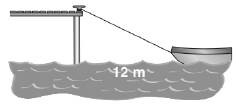Chapter 5.4, Problem 17EElementary Geometry For College St...

7th Edition
Alexander + 2 others
ISBN: 9781337614085

Solutions

Chapter
SectionElementary Geometry For College St...

7th Edition
Alexander + 2 others
ISBN: 9781337614085
Textbook Problem

A boat is 6 m below the level of a pier and 12 m from the pier as measured across the water. How much rope is needed to reach the boat?To determine

To Find:

Length of the rope.

Explanation

Given A boat is 6 m below the level of a pier and 12 m from the pier as measured across the water.

Let x be the length of the rope.

From the figure it is in form of right triangle in which both the sides are 12 m and 6 m.

From the given data,

x2=122+

Still sussing out bartleby?

Check out a sample textbook solution.

See a sample solution

The Solution to Your Study Problems

Bartleby provides explanations to thousands of textbook problems written by our experts, many with advanced degrees!

Get Started

Calculate y'. 50. y=sin2(cossinx)

Single Variable Calculus: Early Transcendentals, Volume I

In Exercises 49-62, find the indicated limit, if it exists. 49. limx1x21x1

Applied Calculus for the Managerial, Life, and Social Sciences: A Brief Approach

Solve for x: e2x1 = 10. a) 12(1+10e) b) 3 + ln 10 c) 2 + ln 10 d) 1+ln102

Study Guide for Stewart's Single Variable Calculus: Early Transcendentals, 8th

For j – 2k 3i – 4k 3i + j – 2k 0 (zero vector)

Study Guide for Stewart's Multivariable Calculus, 8th